Drop a Query

# Jagdeesh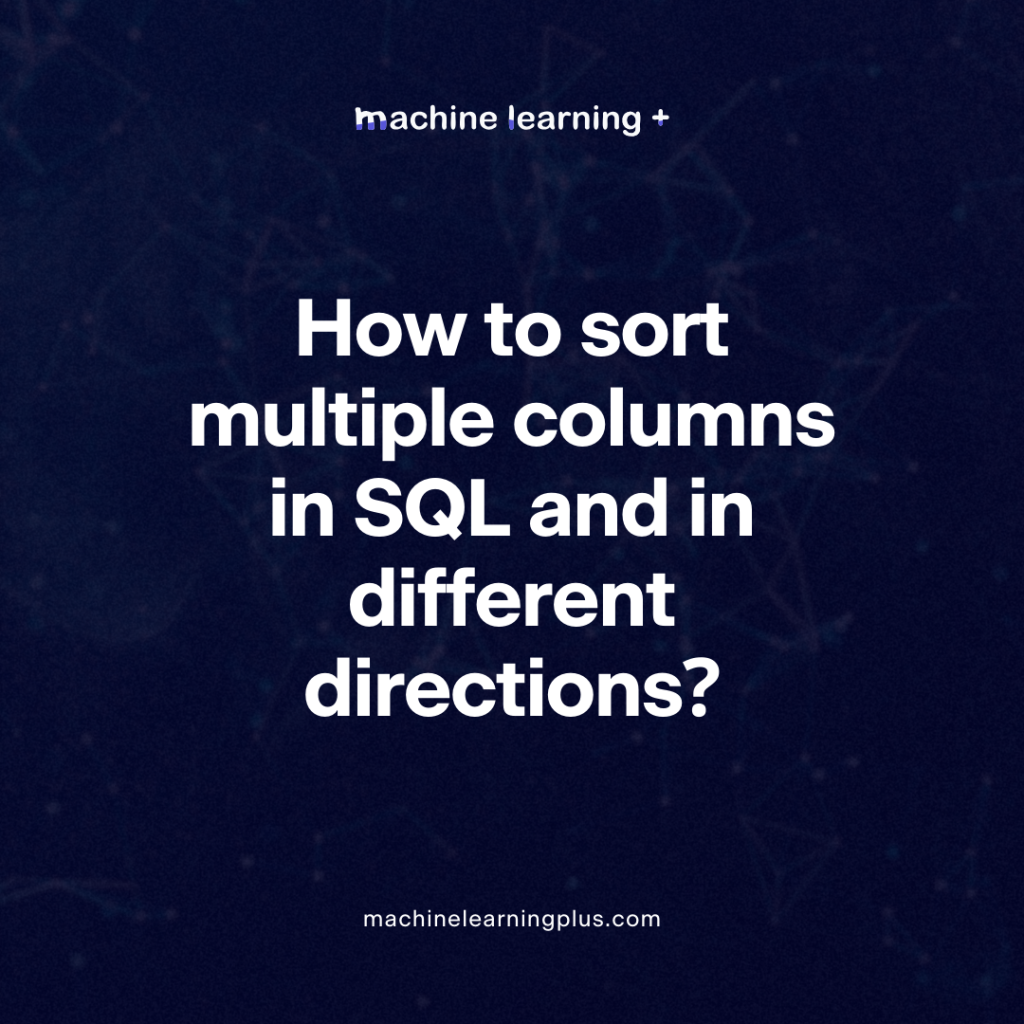## How to sort multiple columns in SQL and in different directions?

Problem How to sort multiple columns in SQL and in different directions? Input Let’s create a table named Employees with columns: id, first_name, last_name, and salary. CREATE TABLE Employees ( id INT PRIMARY KEY AUTO_INCREMENT, first_name VARCHAR(100), last_name VARCHAR(100), salary DECIMAL(10, 2) ); — Insert data into the table INSERT INTO Employees (first_name, last_name, salary) …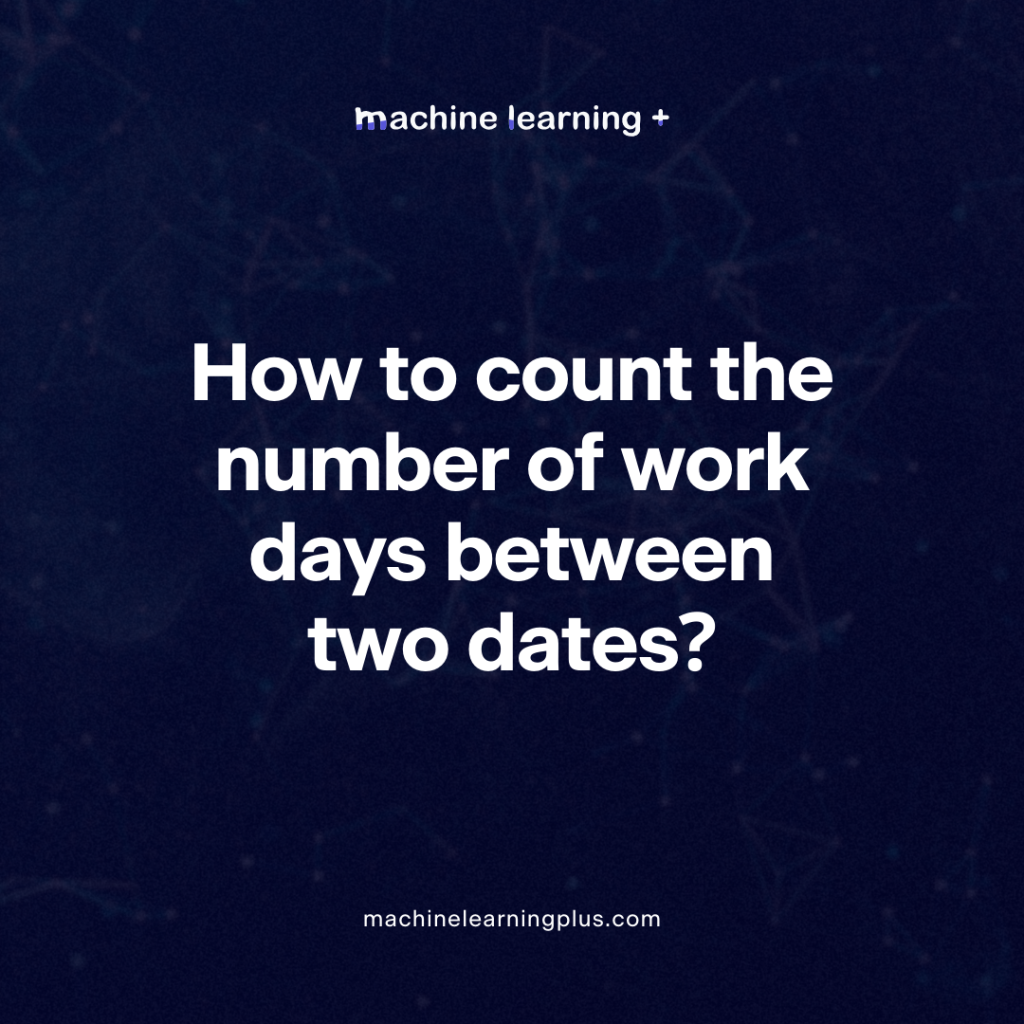## How to count the number of work days between two dates?

Problem To count the number of work days between two dates, taking into account weekends (Saturdays and Sundays) and assuming no holidays. Input We will create a table named DateRange with two columns: StartDate and EndDate. Here’s an example input: CREATE TABLE DateRange ( StartDate DATE, EndDate DATE ); INSERT INTO DateRange(StartDate, EndDate) VALUES (‘2023-10-01’, …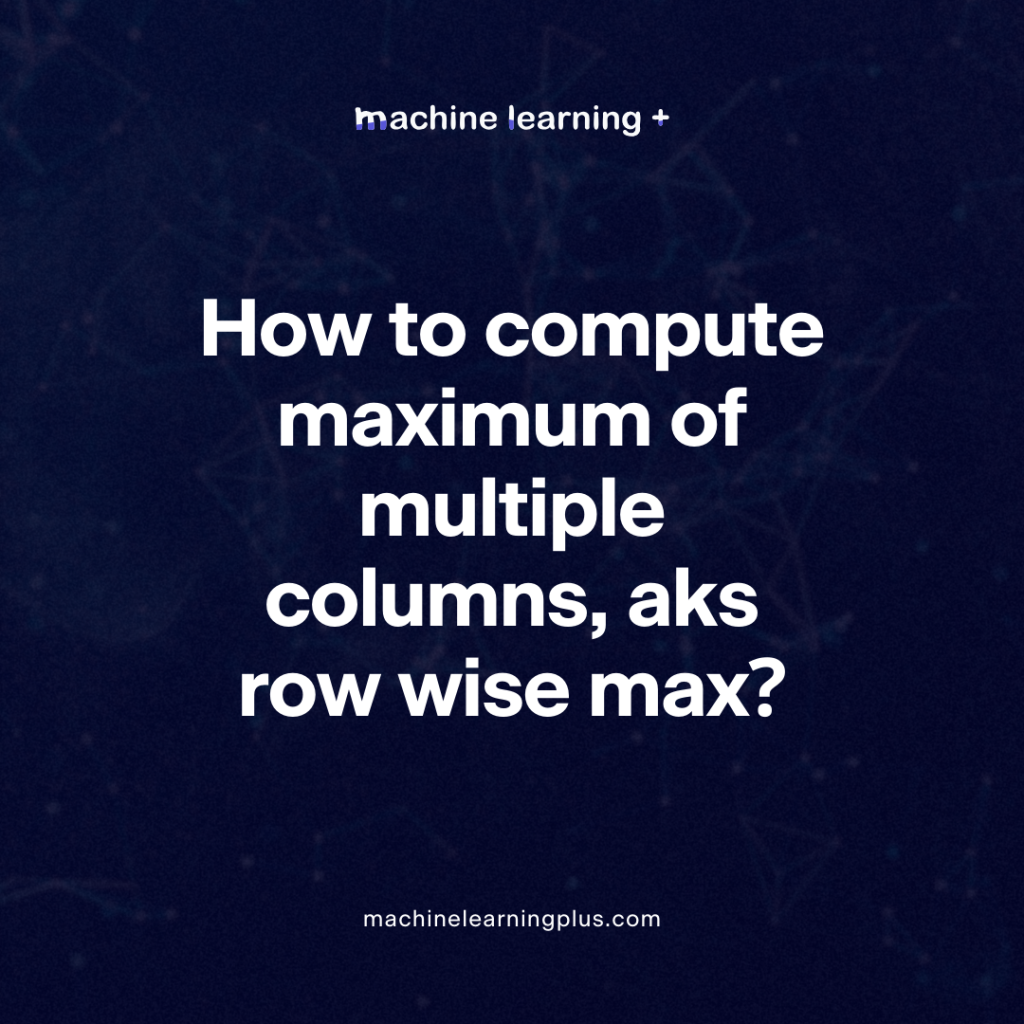## How to compute maximum of multiple columns, aks row wise max?

Problem Given a table with multiple columns, compute the maximum value for each row across the specified columns. Input — Create an example table CREATE TABLE ExampleTable ( ID INT AUTO_INCREMENT PRIMARY KEY, ColumnA INT, ColumnB INT, ColumnC INT ); — Insert data into the table INSERT INTO ExampleTable (ColumnA, ColumnB, ColumnC) VALUES (10, 20, …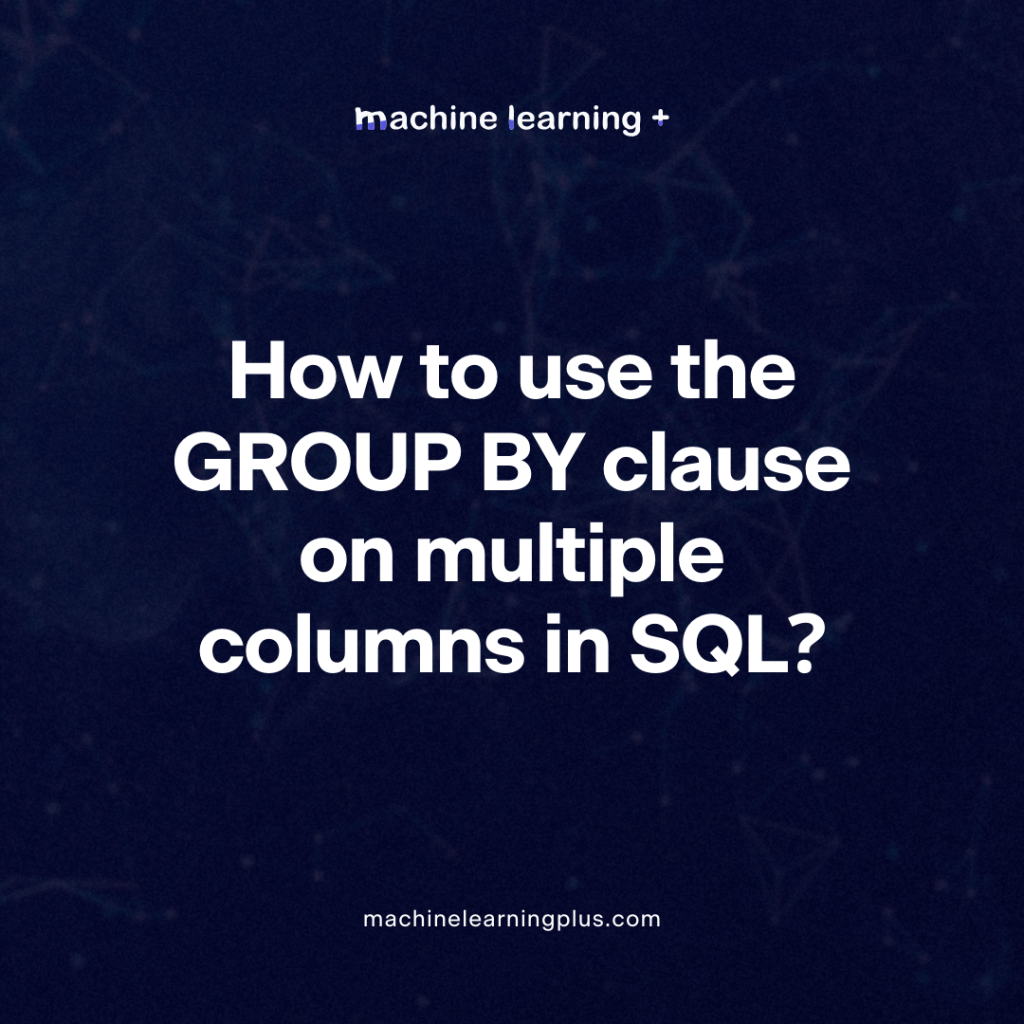## How to use the GROUP BY clause on multiple columns in SQL?

Problem: How to use the GROUP BY clause on multiple columns in SQL? Input: Let’s assume we have a table called Orders with columns OrderID, CustomerID, ProductID, and OrderDate. CREATE TABLE Orders ( OrderID INT PRIMARY KEY, CustomerID INT, ProductID INT, OrderDate DATE ); — Inserting sample data INSERT INTO Orders (OrderID, CustomerID, ProductID, OrderDate) …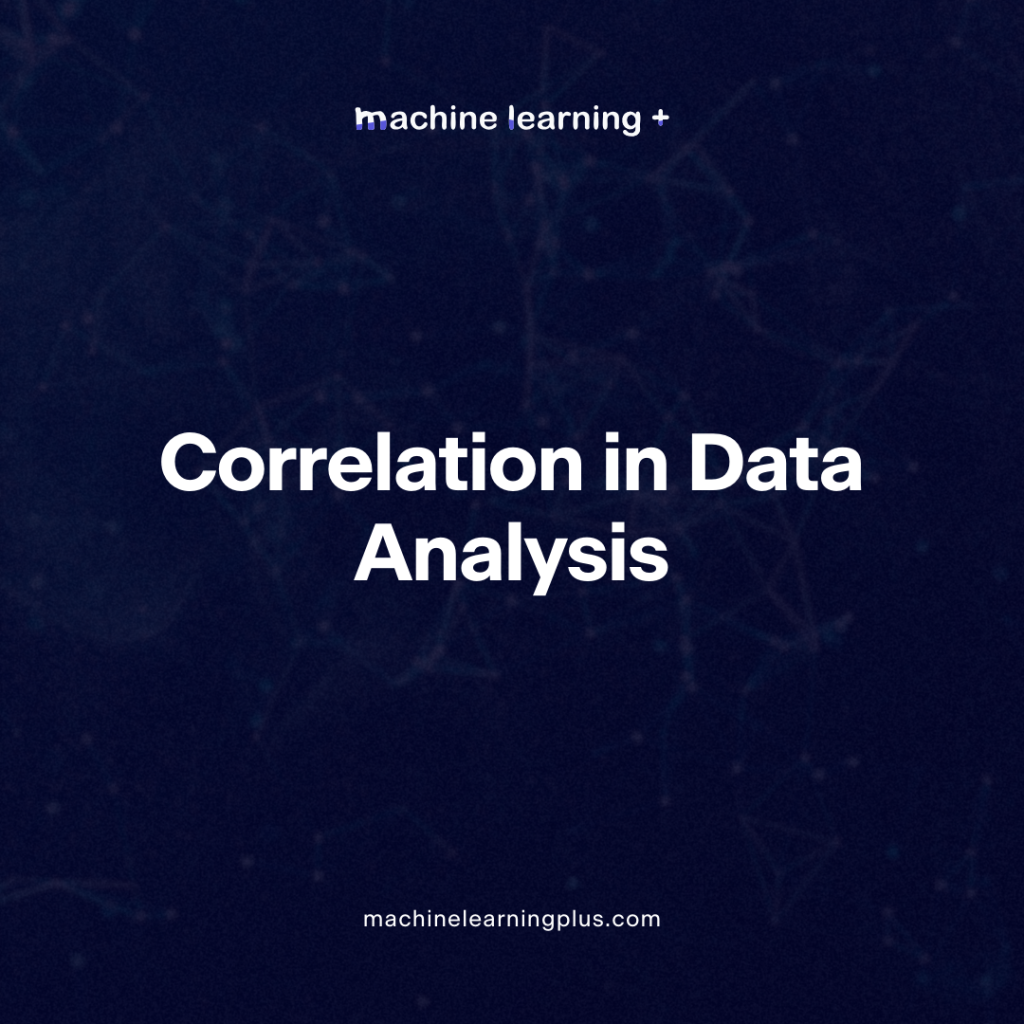## Correlation – Connecting the Dots, the Role of Correlation in Data Analysis

Correlation is a fundamental concept in statistics and data science. It quantifies the degree to which two variables are related. But what does this mean, and how can we use it to our advantage in real-world scenarios? Let’s dive deep into understanding correlation, how to measure it, and its practical implications. In this Blog post …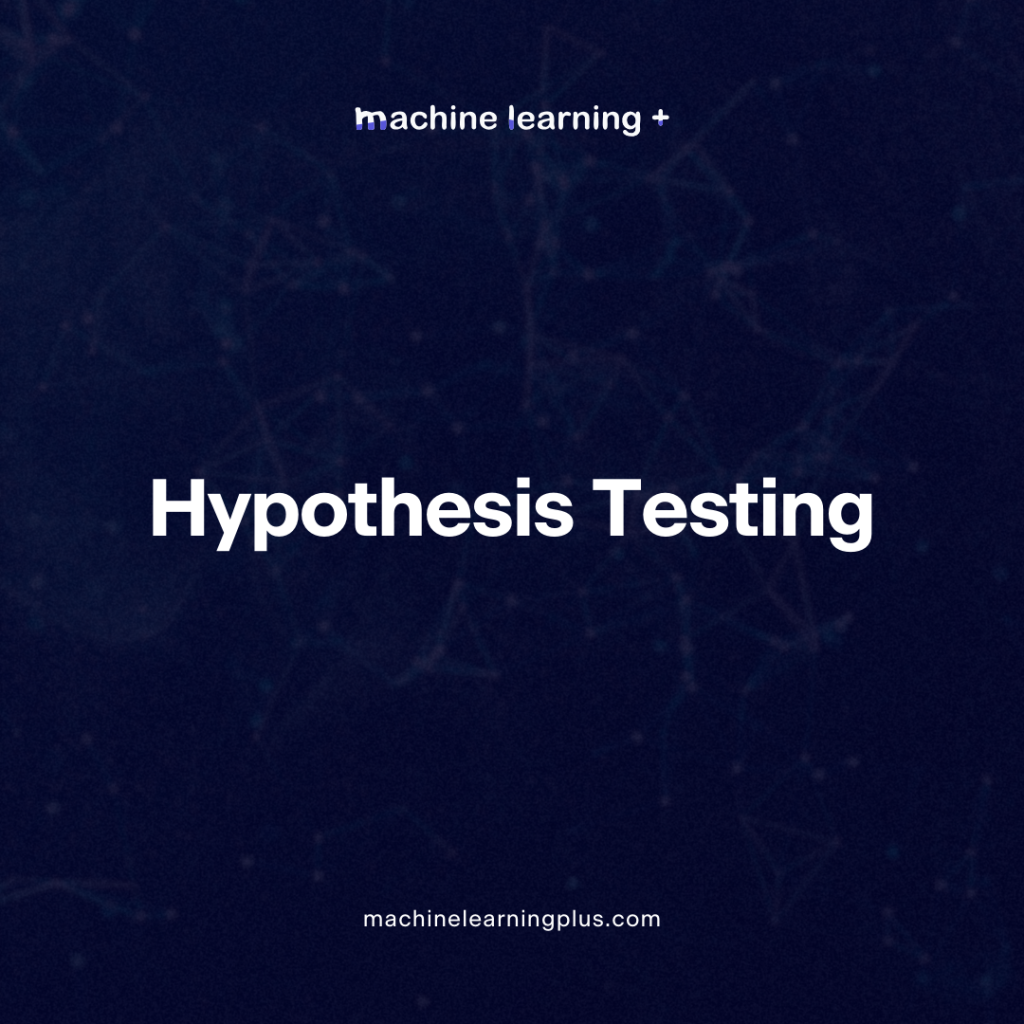## Hypothesis Testing – A Deep Dive into Hypothesis Testing, The Backbone of Statistical Inference

Explore the intricacies of hypothesis testing, a cornerstone of statistical analysis. Dive into methods, interpretations, and applications for making data-driven decisions. In this Blog post we will learn: What is Hypothesis Testing? Steps in Hypothesis Testing 2.1. Set up Hypotheses: Null and Alternative 2.2. Choose a Significance Level (α) 2.3. Calculate a test statistic and …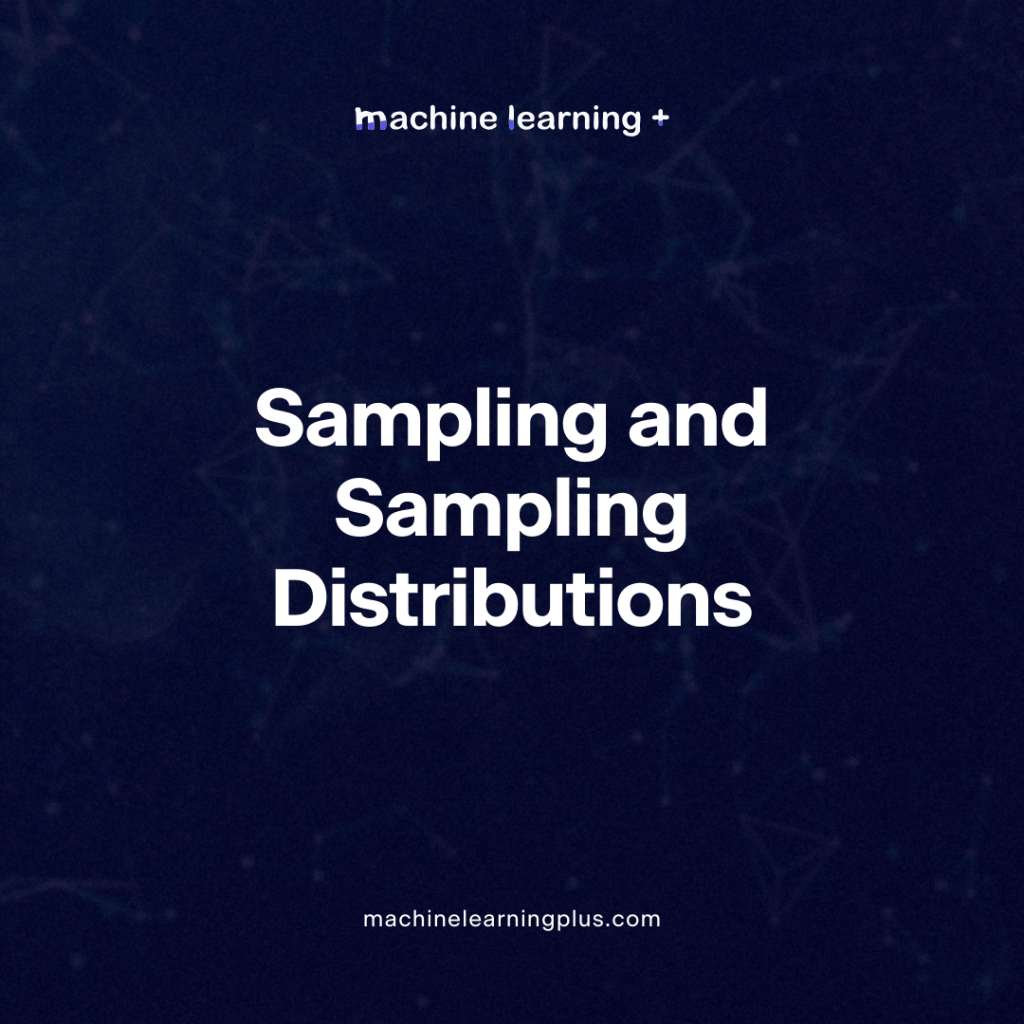## Sampling and Sampling Distributions – A Comprehensive Guide on Sampling and Sampling Distributions

Explore the fundamentals of sampling and sampling distributions in statistics. Dive deep into various sampling methods, from simple random to stratified, and uncover the significance of sampling distributions in detail. In this blog post we will learn What is Sampling? Why Sample? Types of Sampling Methods 3.1. Simple Random Sampling (SRS) 3.2. Stratified Sampling 3.3. …## Law of Large Numbers – A Deep Dive into the World of Statistics

The Law of Large Numbers (LLN) is a fundamental theorem in probability and statistics, serving as the basis for many concepts and practices in the field. If you’ve ever heard the saying “the more the better,” you can think of LLN as the mathematical rendition of this proverb. In this blog post, we’ll dive into …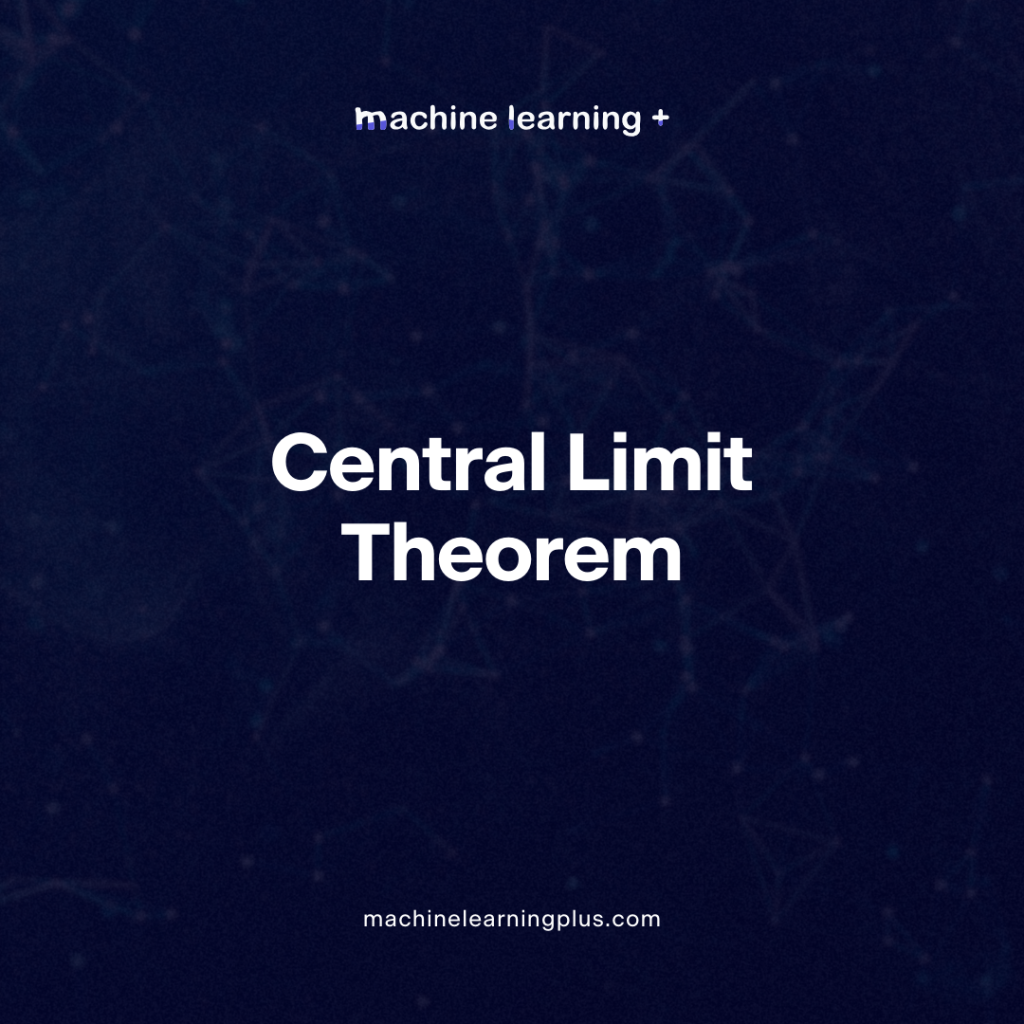## Central Limit Theorem – A Deep Dive into Central Limit Theorem and its Significance in Statistics

Statistics offers a vast array of principles and theorems that are foundational to how we understand data. Among them, the Central Limit Theorem (CLT) stands as one of the most important. Let’s dive deeper into the concept, ensuring that all points are covered and clarified. In this blog post we will learn: Simple Explanation of …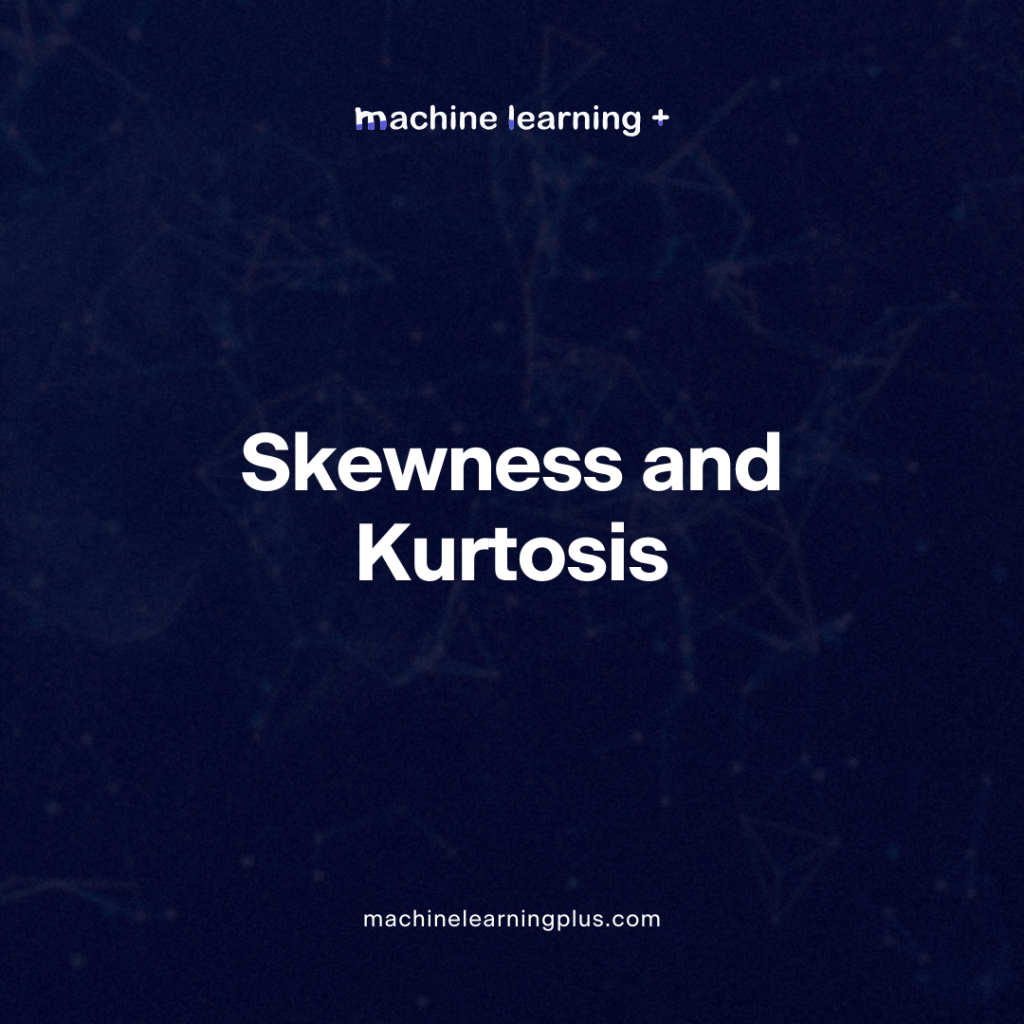## Skewness and Kurtosis – Peaks and Tails, Understanding Data Through Skewness and Kurtosis”

Statistics has a variety of tools to help us understand and interpret data. Two such tools are skewness and kurtosis, which give us insights into the shape of a data distribution. Let’s dive deeper into these concepts and understand their significance. In this blog post we will learn Skewness 1.1. Types of Skewness: 1.2. Rules …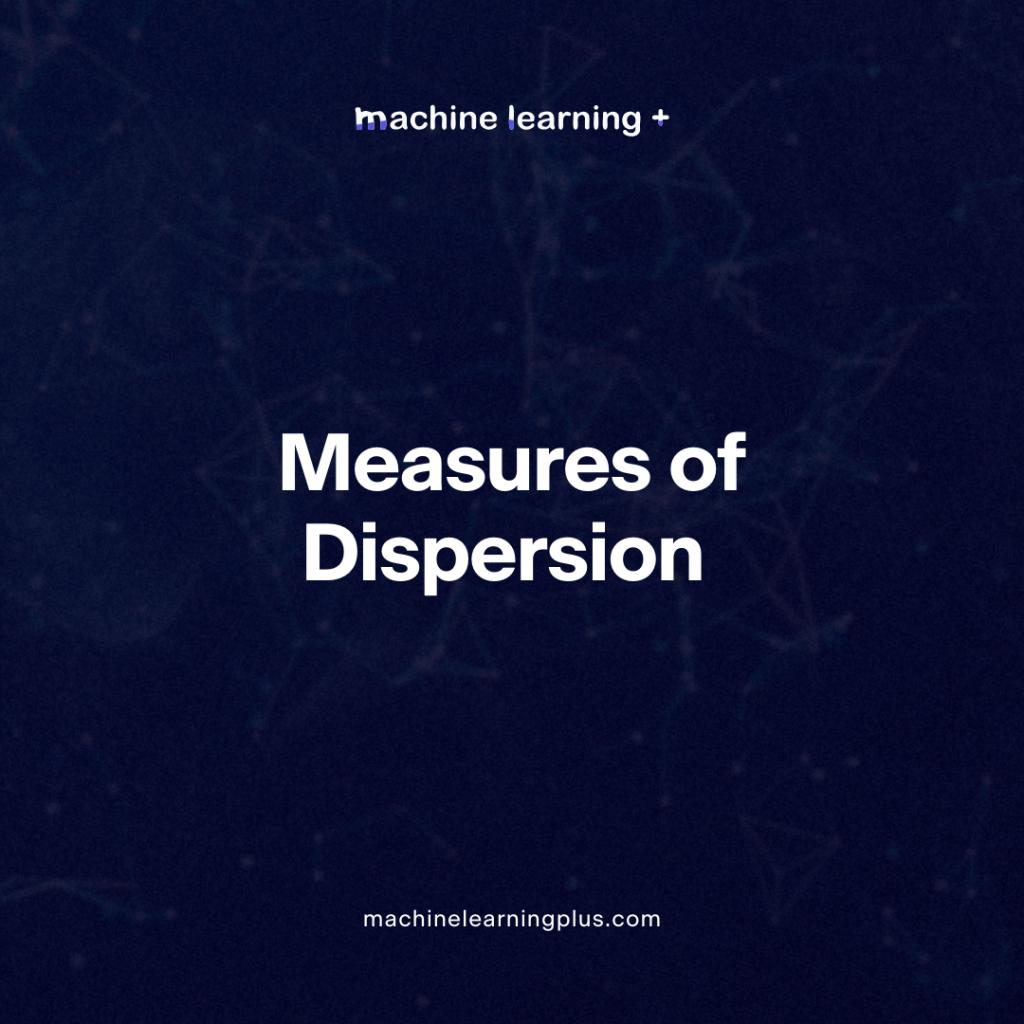## Measures of Dispersion – Unlocking the Variability Diving Deep into Measures of Dispersion

Dive deep into the world of statistics and measures of dispersion, from understanding its essence to its practical application using Python. In this Blog post we will learn: What is Dispersion in Statistics? Advantages and Applications of Measures of Dispersion: Types of Measures of Dispersion 3.1. Absolute Measure of Dispersion 3.2. Relative Measure of Dispersion …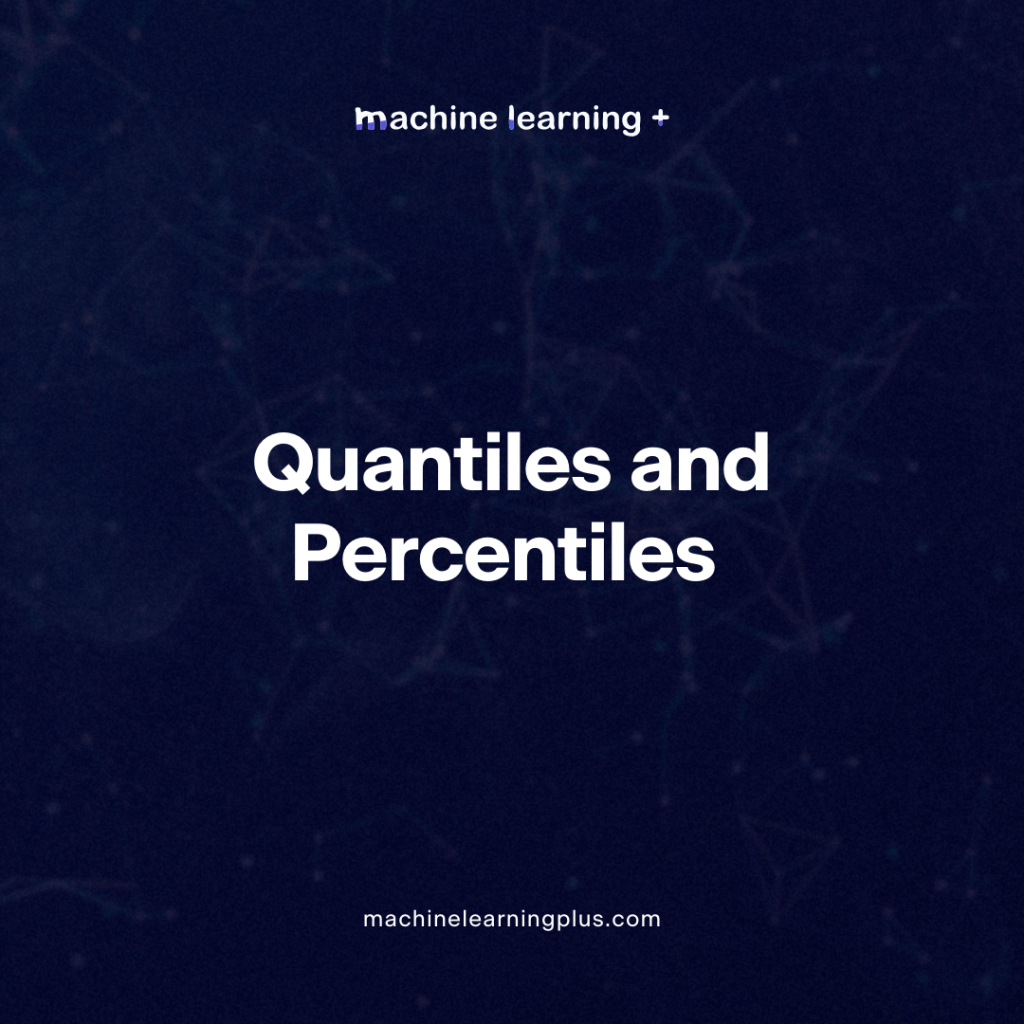## Quantiles and Percentiles – Understanding Quantiles and Percentiles, A Deep Dive with Python Examples

Quantiles and percentiles are crucial statistical concepts that assist in understanding and interpreting data. They are essentially tools to help divide datasets into smaller parts or intervals based on the data’s distribution. Let’s delve deep into these concepts and see them in action with Python. In this blog post we will learn Quantiles Percentiles Why …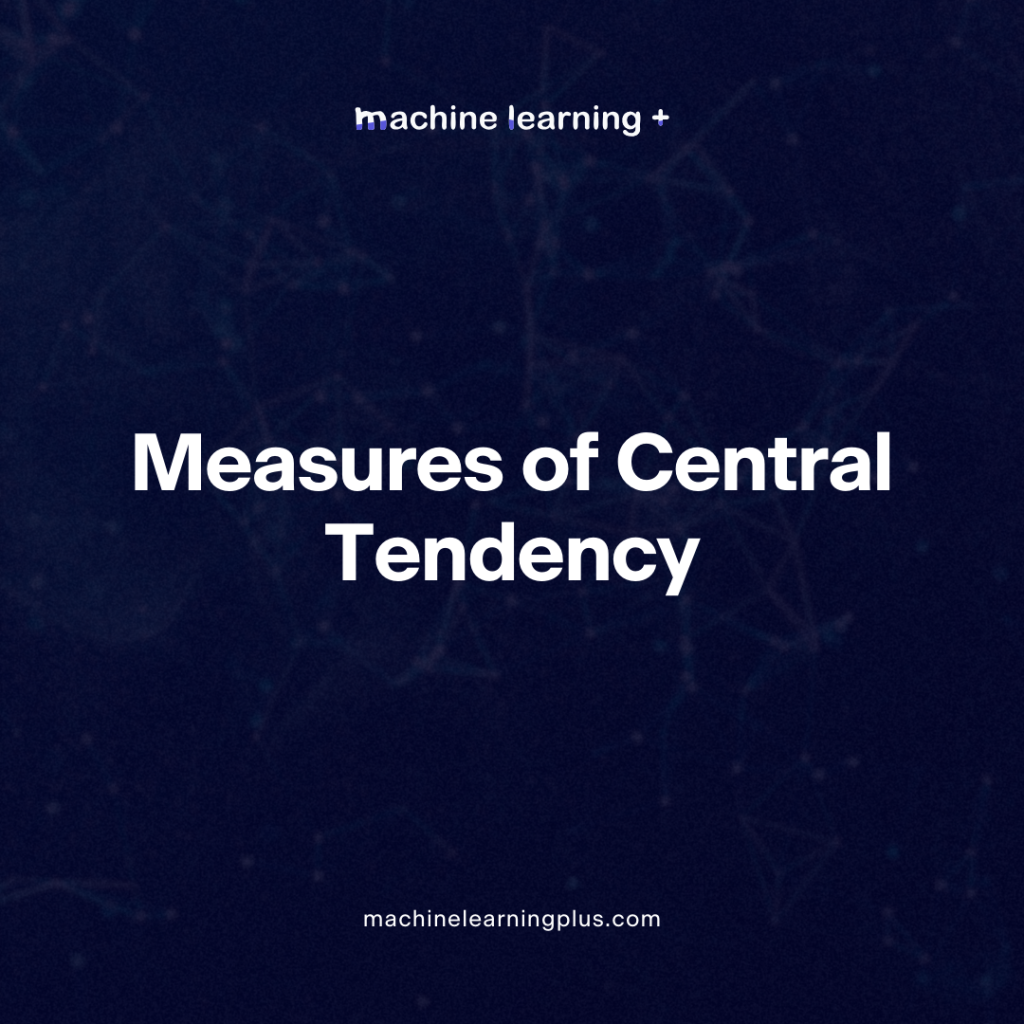## Measures of Central Tendency – A Clear Guide with Examples on Measures of Central Tendency

When diving into the world of statistics, you’ll frequently come across the term “measures of central tendency”. But what exactly does it mean, and why is it so important? Let’s break it down, step by step, with practical examples to drive the point home. In this blog post we will learn: What Are Measures of …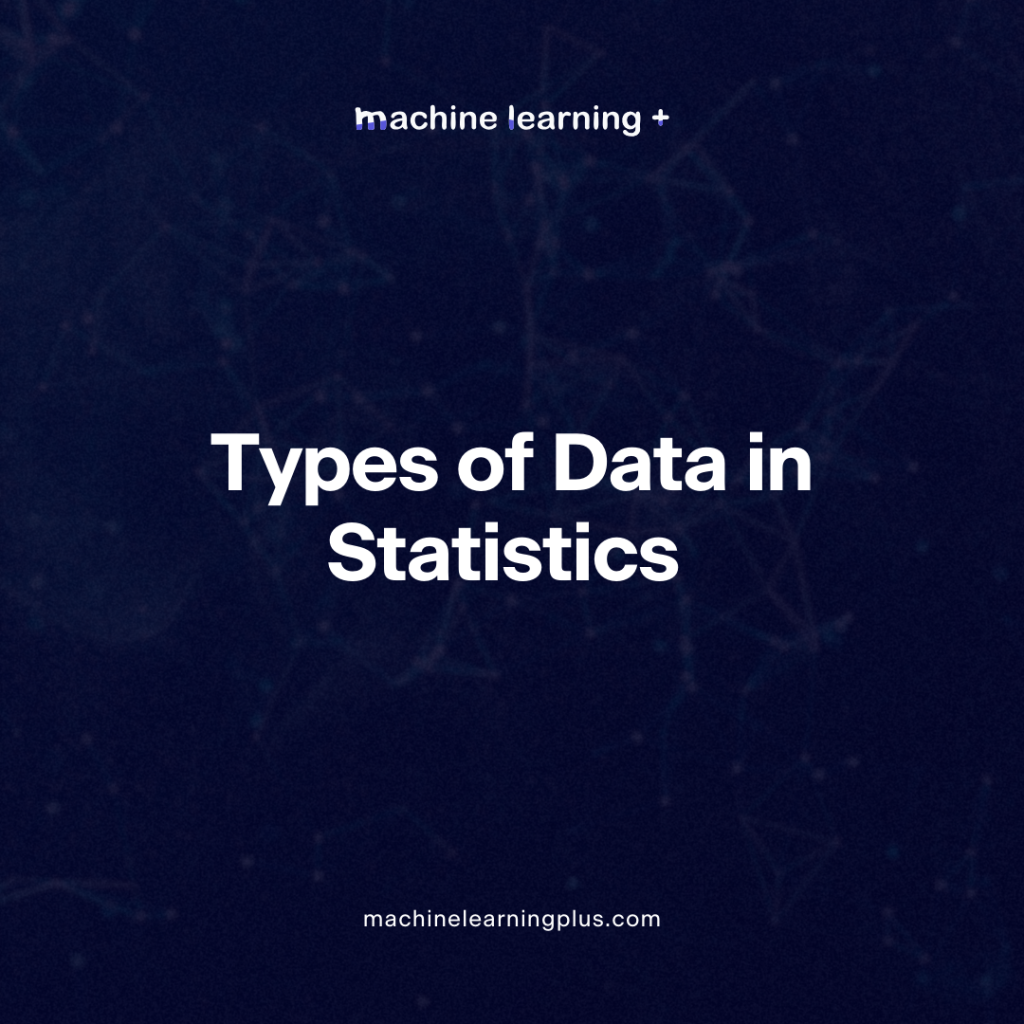## Types of Data in Statistics – A Comprehensive Guide

Statistics is a domain that revolves around the collection, analysis, interpretation, presentation, and organization of data. To appropriately utilize statistical methods and produce meaningful results, understanding the types of data is crucial. In this Blog post we will learn Qualitative Data (Categorical Data) 1.1. Nominal Data: 1.2. Ordinal Data: Quantitative Data (Numerical Data) 2.1. Discrete …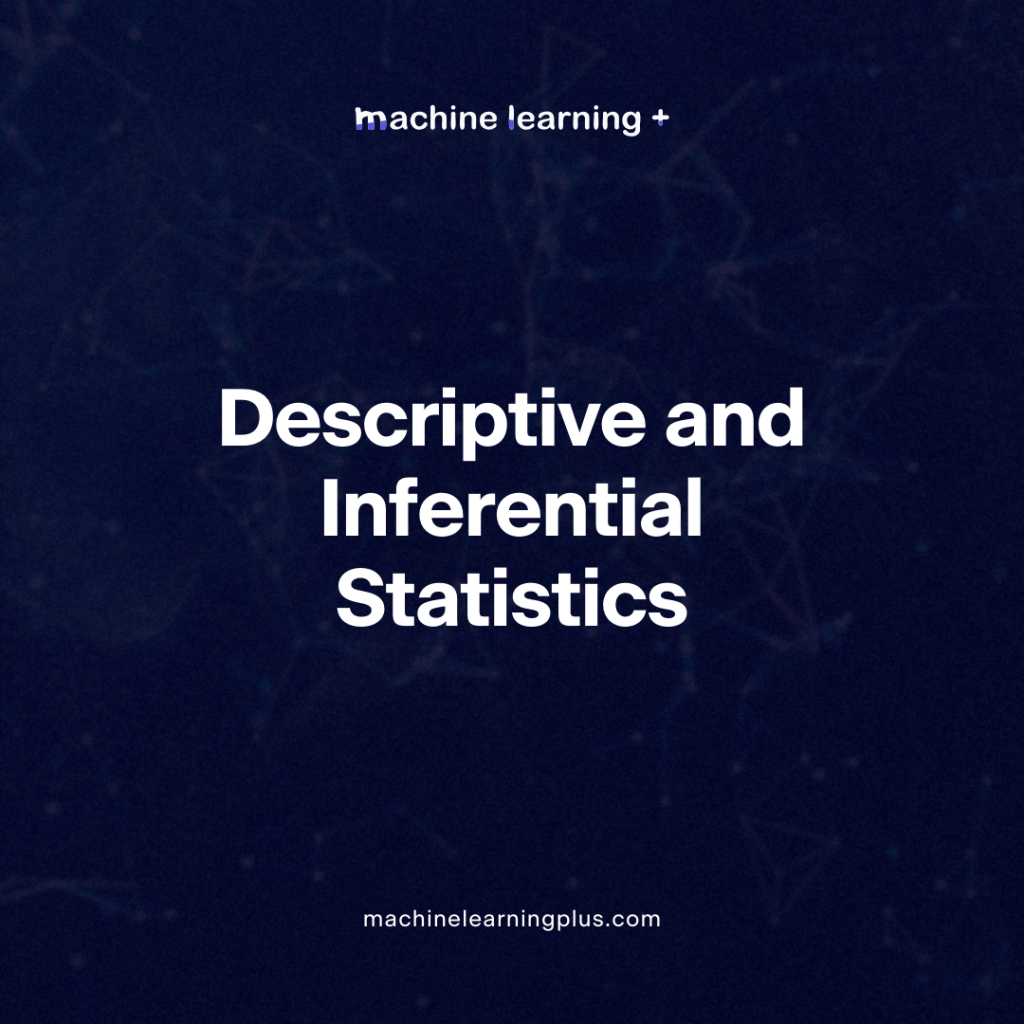## Descriptive and Inferential Statistics – Deep Dive into Descriptive and Inferential Statistics

In statistics understanding the difference between descriptive and inferential statistics is crucial for anyone looking to make sense of data, whether it’s for academic research, business decision-making, or just general curiosity. Let’s dive into these core concepts. In this Blog post we will learn: What is Descriptive Statistics? What is Inferential Statistics? Difference Between Descriptive …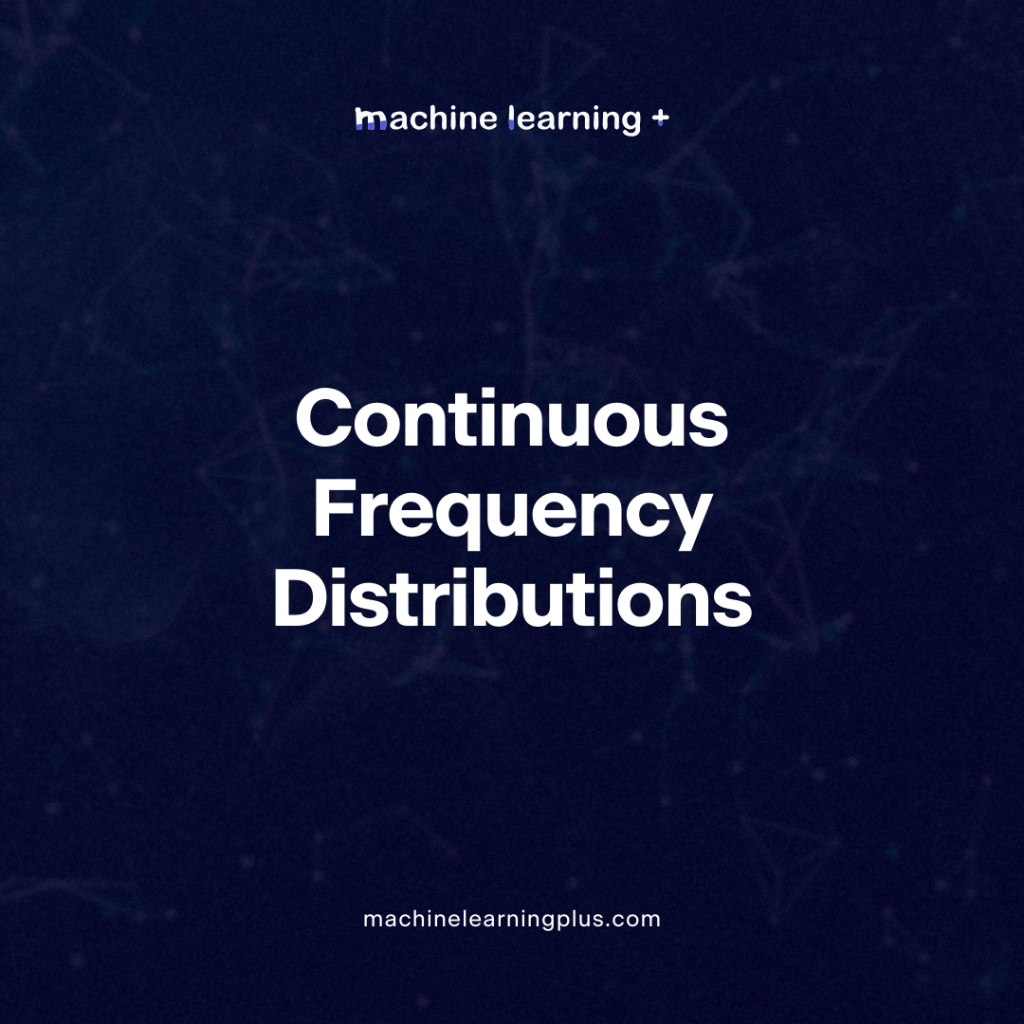## Continuous Frequency Distributions – Understanding Continuous Frequency Distributions and the Probability Density Function (PDF)

Lets deep dive into the world of statistics to understand the mysteries of continuous frequency distributions and the probability density function (PDF). By the end of this post, you’ll have a clearer understanding of these foundational concepts and their significance in the statistics. In this Blog post we will learn: Continuous Frequency Distributions: Constructing the …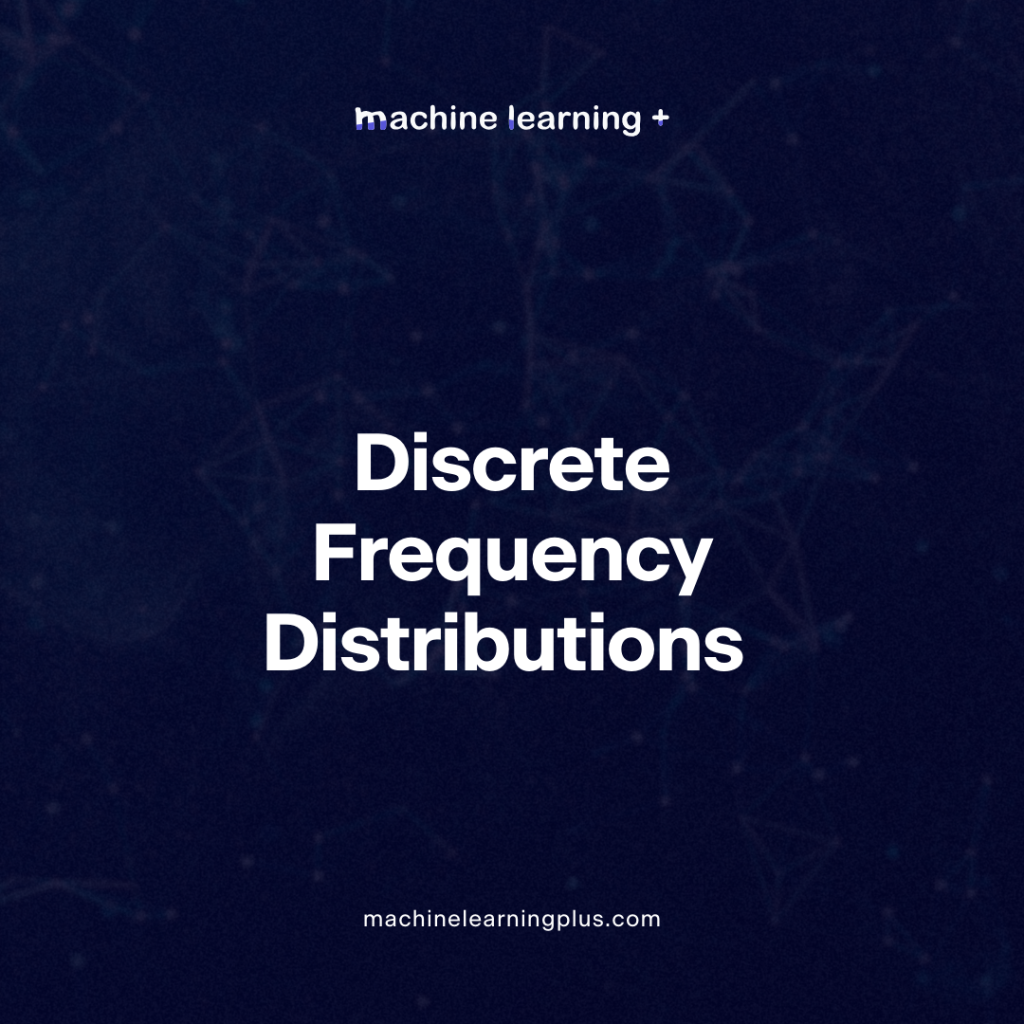## Discrete Frequency Distributions – Decoding Discrete Frequency Distributions and Probability Mass Functions (PMFs) with Real-World Scenarios

Probability and statistics are often viewed as complex areas of study. However, by using real-world examples and some visual aids, the seemingly challenging concepts become quite intuitive. Let’s deep dive into discrete frequency distributions and the different types of Probability Mass Functions (PMFs). In this Blog post we will learn: Discrete Frequency Distribution: Types of …

## Probability frequency distribution – A Comprehensive guide on probability frequency distribution with Examples

A probability frequency distribution is a way to describe the likelihood of an outcome. It gives a clear picture of how often an event can happen relative to all possible outcomes. Whether you’re studying data science, statistics, or merely interested in probability, understanding frequency distribution is crucial. In this Blog post we will learn: Types …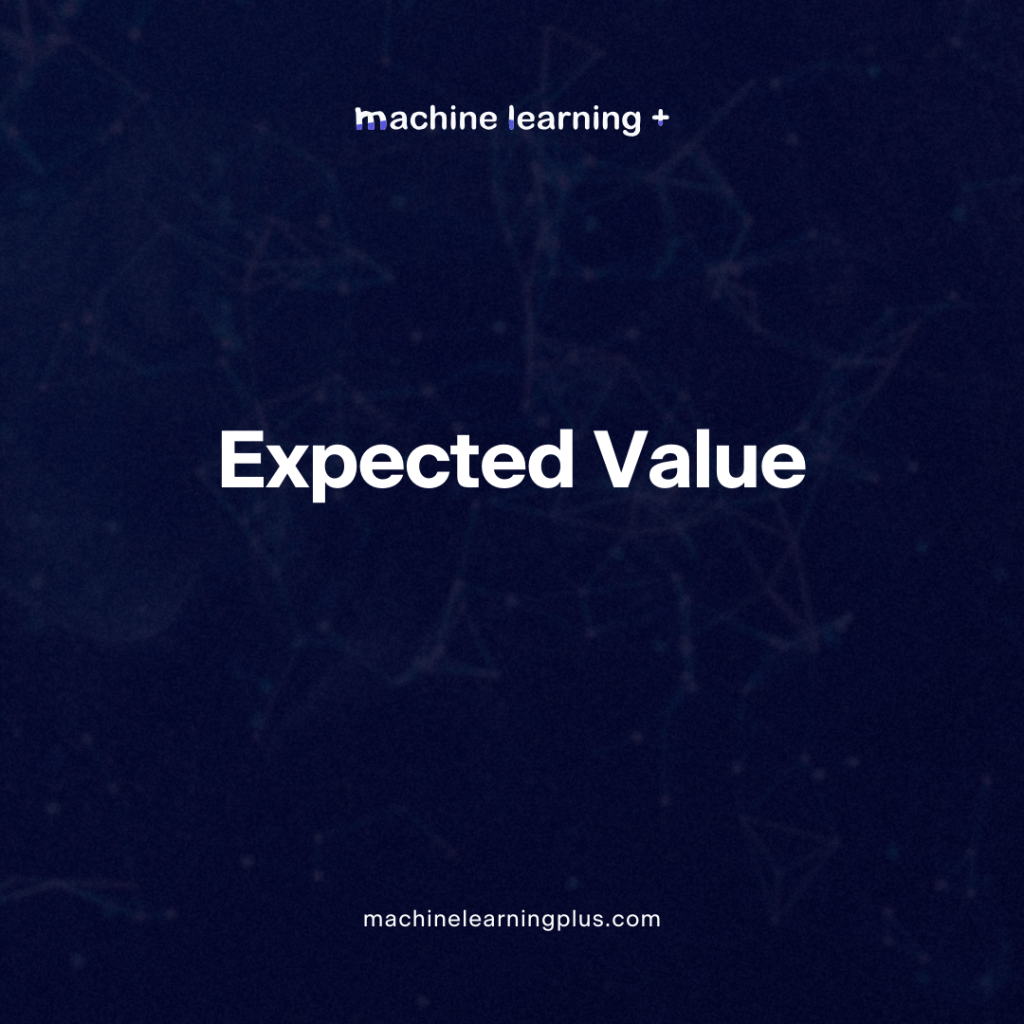## Expected Value – Understanding Expected Value in Probability and Its Real-Time Applications in Machine Learning

In the world of probability and statistics, the concept of the expected value plays a pivotal role. Whether we realize it or not, we make decisions based on expected outcomes in our daily lives. But what does this term mean mathematically, and how is it employed in machine learning? In this Blog post we will …## Bayes’ Theorem – Bayes’ Theorem and Bayesian Inference Unraveling the Mysteries of Probability

Understanding probability can be comparable to navigating a maze. Thankfully, Bayes’ theorem acts as our compass, particularly when we update our predictions with new information. Central to this theorem are three pivotal concepts: the prior, likelihood, and posterior. These elements pave the way for Bayesian inference, where Bayes’ theorem is used to renew the probability …Course Preview

## Machine Learning A-Z™: Hands-On Python & R In Data Science

### Free Sample Videos:#### Machine Learning A-Z™: Hands-On Python & R In Data Science#### Machine Learning A-Z™: Hands-On Python & R In Data Science#### Machine Learning A-Z™: Hands-On Python & R In Data Science#### Machine Learning A-Z™: Hands-On Python & R In Data Science